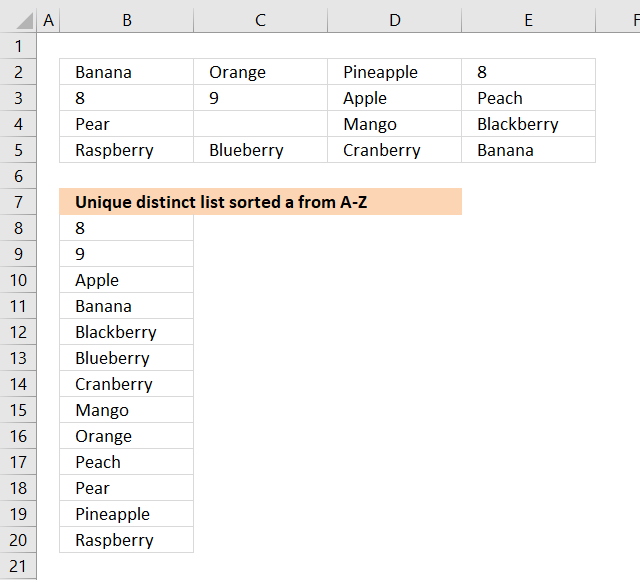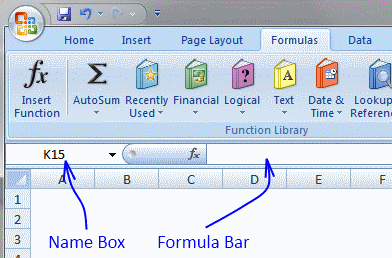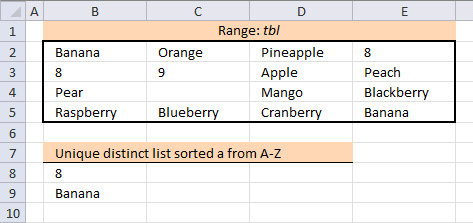Author: Oscar Cronquist Article last updated on January 29, 2019EEK asks:

I am looking for the same formula on this page, but targeting a range of MxN (spanning multiple columns) not just a list in one column.

I see where you have formulas which act on MxN but not with all the features of this one:

1. Unique and distinct
2. Remove blanks
3. Sort
4. Properly handle numbers and text

And just to ask for the 'frosting on top' remove errors.

Answer:

The image above demonstrates an array formula in cell B8 that extracts numbers and text in sorted order, numbers first and then text from A to Z based on cell range. If you want to use a faster way than an array formula then check out Extract unique distinct sorted values from a cell range [UDF].

New shorter array formula in cell B8:

=IFERROR(AGGREGATE(15, 6, tbl/((COUNTIF(\$B\$7:B7, tbl)=0)*(tbl<>"")), 1), TEXTJOIN("", TRUE, IF(MIN(IF(SMALL(IF(ISTEXT(tbl)*(COUNTIF(\$B\$7:B7, tbl&"")=0), COUNTIF(tbl, "<"&tbl), ""), 1)=IFERROR(COUNTIF(tbl, "<"&tbl)/ISTEXT(tbl), ""), (ROW(tbl)+(1/(COLUMN(tbl)+1)))*1, ""))=(ROW(tbl)+(1/(COLUMN(tbl)+1)))*1, tbl, "")))

To enter an array formula, type the formula in a cell then press and hold CTRL + SHIFT simultaneously, now press Enter once. Release all keys.

The formula bar now shows the formula with a beginning and ending curly bracket telling you that you entered the formula successfully. Don't enter the curly brackets yourself.

You need to customize the formula so it suits your worksheet. It is easy, simply replace all instances of \$B\$7:B7 in the formula above with a cell reference to the cell right above the cell you enter the formula in in your worksheet.

Example, you are about to enter the formula in cell F7 in your worksheet, you now need to replace \$B\$7:B7 with a reference to the cell right above cell F7 and that is F6, however, it must look like this: \$F\$6:F6. It is a growing cell reference that will expand automatically when you copy cell F7 and paste to cells below, I will explain it in greater detail below.

Named range

The formula above contains a named range tbl, it is simply a reference to a cell range and they are easily and quickly created.

1. Select cell range B2:E5.
2. Type tbl in Name Box.### Explaining new formula in cell B8

There are two parts in the new array formula above, the first part extracts numbers from the cell range and the second part extracts text values.

The IFERROR function moves from the first part of the formula to the second part as soon as an error is detected in the first part. An error is created in the first part when there are no more numbers to extract.

IFERROR( formula_part1, formula_part2)

#### Step 1 - Identify previous values

The COUNTIF function counts values based on a condition or criteria, the first argument contains an expanding cell reference, it grows when the cell is copied to the cells below. This makes the formula aware of values displayed in cells above. 0 (zero) indicates values that not yet have been displayed

COUNTIF(\$B\$7:B7, tbl)=0

becomes

COUNTIF(\$B\$7:B7, B2:E5)=0

becomes

{0,0,0,0;0,0,0,0;0,0,0,0;0,0,0,0}=0

and returns

{TRUE, TRUE, TRUE, TRUE;TRUE, TRUE, TRUE, TRUE;TRUE, TRUE, TRUE, TRUE;TRUE, TRUE, TRUE, TRUE}

#### Step 2 - Find not empty cells

The less than and larger than sign combined is interpreted as not equal to by Excel. B2:E5<>"" means

tbl<>""

becomes

B2:E5<>""

and returns

{TRUE, TRUE, TRUE, TRUE;TRUE, TRUE, TRUE, TRUE;TRUE, FALSE, TRUE, TRUE;TRUE, TRUE, TRUE, TRUE}

This array contains boolean values indicating if cell is empty (FALSE) or not empty (TRUE).

#### Step 3 - Divide numbers with array

tbl/((COUNTIF(\$B\$7:B7, tbl)=0)*(tbl<>""))

becomes

{"Banana", "Orange", "Pineapple", 8;8, 9, "Apple", "Peach";"Pear", 0, "Mango", "Blackberry";"Raspberry", "Blueberry", "Cranberry", "Banana"}/((COUNTIF(\$B\$7:B7, tbl)=0)*(tbl<>""))

becomes

{"Banana", "Orange", "Pineapple", 8;8, 9, "Apple", "Peach";"Pear", 0, "Mango", "Blackberry";"Raspberry", "Blueberry", "Cranberry", "Banana"}/({TRUE, TRUE, TRUE, TRUE;TRUE, TRUE, TRUE, TRUE;TRUE, TRUE, TRUE, TRUE;TRUE, TRUE, TRUE, TRUE}*{TRUE, TRUE, TRUE, TRUE;TRUE, TRUE, TRUE, TRUE;TRUE, FALSE, TRUE, TRUE;TRUE, TRUE, TRUE, TRUE})

becomes

{"Banana", "Orange", "Pineapple", 8;8, 9, "Apple", "Peach";"Pear", 0, "Mango", "Blackberry";"Raspberry", "Blueberry", "Cranberry", "Banana"}/{1,1,1,1;1,1,1,1;1,0,1,1;1,1,1,1}

and returns

{#VALUE!, #VALUE!, #VALUE!, 8; 8, 9, #VALUE!, #VALUE!; #VALUE!, #DIV/0!, #VALUE!, #VALUE!; #VALUE!, #VALUE!, #VALUE!, #VALUE!}

#### Step 4 - Extract k-th smallest number in array

The AGGREGATE function lets you extract the k-th smallest number ignoring error values.

AGGREGATE(15, 6, tbl/((COUNTIF(\$B\$7:B7, tbl)=0)*(tbl<>"")), 1)

becomes

AGGREGATE(15, 6, {#VALUE!, #VALUE!, #VALUE!, 8; 8, 9, #VALUE!, #VALUE!; #VALUE!, #DIV/0!, #VALUE!, #VALUE!; #VALUE!, #VALUE!, #VALUE!, #VALUE!}, 1)

and returns 8 in cell B8.

#### Step 1 - Explaining second part of formula in cell B10

The following steps explain how to extract text values sorted from A to Z ignoring blanks. This step extracts a number indicating the alphabetical sort order of each text value.

Note that it is the formula in cell B10 I am explaining now. The ISTEXT function returns TRUE if the cell contains a text value.

COUNTIF(tbl, "<"&tbl) returns an array containing numbers representing the alphabetical sort order of each text value.

COUNTIF(\$B\$7:B7, tbl&"") makes sure that values displayed above current cell is ignored, we don't want to extract duplicate values.

IF(ISTEXT(tbl)*(COUNTIF(\$B\$7:B7, tbl&"")=0), COUNTIF(tbl, "<"&tbl), "")

becomes

IF({TRUE, TRUE, TRUE, FALSE; FALSE, FALSE, TRUE, TRUE; TRUE, FALSE, TRUE, TRUE; TRUE, TRUE, TRUE, TRUE}*(COUNTIF(\$B\$7:B7, tbl&"")=0), COUNTIF(tbl, "<"&tbl), "")

becomes

IF({TRUE, TRUE, TRUE, FALSE; FALSE, FALSE, TRUE, TRUE; TRUE, FALSE, TRUE, TRUE; TRUE, TRUE, TRUE, TRUE}*{TRUE, TRUE, TRUE, FALSE;FALSE, FALSE, TRUE, TRUE;TRUE, TRUE, TRUE, TRUE;TRUE, TRUE, TRUE, TRUE}, COUNTIF(tbl, "<"&tbl), "")

becomes

IF({1,1,1,0;0,0,1,1;1,0,1,1;1,1,1,1}, {1, 7, 10, 0;0, 2, 0, 8;9, 0, 6, 3;11, 4, 5, 1}, "")

and returns

{1, 7, 10, "";"", "", 0, 8;9, "", 6, 3;11, 4, 5, 1}

#### Step 2 - Find smallest number and return a unique number based on row and column

The SMALL function extracts the smallest value in the array. The IF function compares the smallest number to the array in order to identify where the value is located.

COUNTIF(tbl, "<"&tbl)/ISTEXT(tbl) returns !DIV/0 errors for all numbers in the cell range, we want to compare text values only.

(ROW(tbl)+(1/(COLUMN(tbl)+1)))*1 returns unique numbers for each value in cell range, we must avoid duplicate values in the array.

IF(SMALL(IF(ISTEXT(tbl)*(COUNTIF(\$B\$7:B7, tbl&"")=0), COUNTIF(tbl, "<"&tbl), ""), 1)=IFERROR(COUNTIF(tbl, "<"&tbl)/ISTEXT(tbl), ""), (ROW(tbl)+(1/(COLUMN(tbl)+1)))*1, "")

becomes

IF(SMALL({1, 7, 10, "";"", "", 0, 8;9, "", 6, 3;11, 4, 5, 1}, 1)=IFERROR(COUNTIF(tbl, "<"&tbl)/ISTEXT(tbl), ""), (ROW(tbl)+(1/(COLUMN(tbl)+1)))*1, "")

becomes

IF(0=IFERROR(COUNTIF(tbl, "<"&tbl)/ISTEXT(tbl), ""), (ROW(tbl)+(1/(COLUMN(tbl)+1)))*1, "")

becomes

IF(0=IFERROR({1,7,10,0;0,2,0,8;9,0,6,3;11,4,5,1}/ISTEXT(tbl), ""), (ROW(tbl)+(1/(COLUMN(tbl)+1)))*1, "")

becomes

IF(0=IFERROR({1,7,10,0;0,2,0,8;9,0,6,3;11,4,5,1}/{TRUE, TRUE, TRUE, FALSE; FALSE, FALSE, TRUE, TRUE; TRUE, FALSE, TRUE, TRUE; TRUE, TRUE, TRUE, TRUE}, ""), (ROW(tbl)+(1/(COLUMN(tbl)+1)))*1, "")

becomes

IF(0=IFERROR({1,7,10,0;0,2,0,8;9,0,6,3;11,4,5,1}/{TRUE, TRUE, TRUE, FALSE; FALSE, FALSE, TRUE, TRUE; TRUE, FALSE, TRUE, TRUE; TRUE, TRUE, TRUE, TRUE}, ""), (ROW(tbl)+(1/(COLUMN(tbl)+1)))*1, "")

becomes

IF(0=IFERROR({1,7,10,#DIV/0!;#DIV/0!,#DIV/0!,0,8;9,#DIV/0!,6,3;11,4,5,1}, ""), (ROW(tbl)+(1/(COLUMN(tbl)+1)))*1, "")

becomes

IF(0={1,7,10,"";"","",0,8;9,"",6,3;11,4,5,1}, (ROW(tbl)+(1/(COLUMN(tbl)+1)))*1, "")

becomes

IF({FALSE, FALSE, FALSE, FALSE;FALSE, FALSE, TRUE, FALSE;FALSE, FALSE, FALSE, FALSE;FALSE, FALSE, FALSE, FALSE}, (ROW(tbl)+(1/(COLUMN(tbl)+1)))*1, "")

becomes

IF({FALSE, FALSE, FALSE, FALSE;FALSE, FALSE, TRUE, FALSE;FALSE, FALSE, FALSE, FALSE;FALSE, FALSE, FALSE, FALSE}, {2.33333333333333, 2.25, 2.2, 2.16666666666667;3.33333333333333, 3.25, 3.2, 3.16666666666667;4.33333333333333, 4.25, 4.2, 4.16666666666667;5.33333333333333, 5.25, 5.2, 5.16666666666667}, "")

and returns

{"","","","";"","",3.2,"";"","","","";"","","",""}

#### Step 3 - Extract value based on unique number

IF(MIN(IF(SMALL(IF(ISTEXT(tbl)*(COUNTIF(\$B\$7:B7, tbl&"")=0), COUNTIF(tbl, "<"&tbl), ""), 1)=IFERROR(COUNTIF(tbl, "<"&tbl)/ISTEXT(tbl), ""), (ROW(tbl)+(1/(COLUMN(tbl)+1)))*1, ""))=(ROW(tbl)+(1/(COLUMN(tbl)+1)))*1, tbl, "")

becomes

IF(MIN({"","","","";"","",3.2,"";"","","","";"","","",""})=(ROW(tbl)+(1/(COLUMN(tbl)+1)))*1, tbl, "")

becomes

IF(3.2=(ROW(tbl)+(1/(COLUMN(tbl)+1)))*1, tbl, "")

becomes

IF(3.2={2.33333333333333, 2.25, 2.2, 2.16666666666667;3.33333333333333, 3.25, 3.2, 3.16666666666667;4.33333333333333, 4.25, 4.2, 4.16666666666667;5.33333333333333, 5.25, 5.2, 5.16666666666667}, tbl, "")

becomes

IF({FALSE, FALSE, FALSE, FALSE;FALSE, FALSE, TRUE, FALSE;FALSE, FALSE, FALSE, FALSE;FALSE, FALSE, FALSE, FALSE}, tbl, "")

becomes

IF({FALSE, FALSE, FALSE, FALSE;FALSE, FALSE, TRUE, FALSE;FALSE, FALSE, FALSE, FALSE;FALSE, FALSE, FALSE, FALSE}, {"Banana", "Orange", "Pineapple", 8;8, 9, "Apple", "Peach";"Pear", 0, "Mango", "Blackberry";"Raspberry", "Blueberry", "Cranberry", "Banana"}, "")

and returns

{"","","","";"","","Apple","";"","","","";"","","",""}

#### Step 4 - Concatenate strings ignoring blanks

The TEXTJOIN function concatenates values ignoring blanks.

TEXTJOIN("", TRUE, IF(MIN(IF(SMALL(IF(ISTEXT(tbl)*(COUNTIF(\$B\$7:B7, tbl&"")=0), COUNTIF(tbl, "<"&tbl), ""), 1)=IFERROR(COUNTIF(tbl, "<"&tbl)/ISTEXT(tbl), ""), (ROW(tbl)+(1/(COLUMN(tbl)+1)))*1, ""))=(ROW(tbl)+(1/(COLUMN(tbl)+1)))*1, tbl, ""))

becomes

TEXTJOIN("", TRUE, {"","","","";"","","Apple","";"","","","";"","","",""})

and returns "Apple" in cell B8.

Old Excel 2007/2010 array formula in cell B8:

=IFERROR(SMALL(IF((tbl<>"")*(ISNUMBER(tbl))*(COUNTIF(\$B\$7:B7, tbl)=0), tbl, ""), 1), IFERROR(INDEX(tbl, SMALL(IF(SMALL(IF((COUNTIF(\$B\$7:B7, tbl)=0)*(ISTEXT(tbl)), COUNTIF(tbl, "<"&tbl)+1, ""), 1)=IF((COUNTIF(\$B\$7:B7, tbl)=0)*(ISTEXT(tbl)), COUNTIF(tbl, "<"&tbl)+1, ""), ROW(tbl)-MIN(ROW(tbl))+1), 1), MATCH(SMALL(IF((COUNTIF(\$B\$7:B7, tbl)=0)*(ISTEXT(tbl)), COUNTIF(tbl, "<"&tbl)+1, ""), 1), INDEX(IF((COUNTIF(\$B\$7:B7, tbl)=0)*(ISTEXT(tbl)), COUNTIF(tbl, "<"&tbl)+1, ""), SMALL(IF(SMALL(IF((COUNTIF(\$B\$7:B7, tbl)=0)*(ISTEXT(tbl)), COUNTIF(tbl, "<"&tbl)+1, ""), 1)=IF((COUNTIF(\$B\$7:B7, tbl)=0)*(ISTEXT(tbl)), COUNTIF(tbl, "<"&tbl)+1, ""), ROW(tbl)-MIN(ROW(tbl))+1), 1), , 1), 0), 1), ""))

### Download Excel file

* You will also get a weekly newsletter, unsubscribe anytime!

### Excel 2007/2010 array formula: Filter duplicate values, sorted and blanks removedArray formula in cell B8:

=IFERROR(SMALL(IF((tbl<>"")*(ISNUMBER(tbl))*(COUNTIF(\$B\$7:B7, tbl)=0)*(COUNTIF(tbl, tbl)>1), tbl, ""), 1), IFERROR(INDEX(tbl, SMALL(IF(SMALL(IF((COUNTIF(\$B\$7:B7, tbl)=0)*(ISTEXT(tbl)*(COUNTIF(tbl, tbl)>1)), COUNTIF(tbl, "<"&tbl)+1, ""), 1)=IF((COUNTIF(\$B\$7:B7, tbl)=0)*(ISTEXT(tbl)*(COUNTIF(tbl, tbl)>1)), COUNTIF(tbl, "<"&tbl)+1, ""), ROW(tbl)-MIN(ROW(tbl))+1), 1), MATCH(SMALL(IF((COUNTIF(\$B\$7:B7, tbl)=0)*(ISTEXT(tbl)*(COUNTIF(tbl, tbl)>1)), COUNTIF(tbl, "<"&tbl)+1, ""), 1), INDEX(IF((COUNTIF(\$B\$7:B7, tbl)=0)*(ISTEXT(tbl)*(COUNTIF(tbl, tbl)>1)), COUNTIF(tbl, "<"&tbl)+1, ""), SMALL(IF(SMALL(IF((COUNTIF(\$B\$7:B7, tbl)=0)*(ISTEXT(tbl)*(COUNTIF(tbl, tbl)>1)), COUNTIF(tbl, "<"&tbl)+1, ""), 1)=IF((COUNTIF(\$B\$7:B7, tbl)=0)*(ISTEXT(tbl)*(COUNTIF(tbl, tbl)>1)), COUNTIF(tbl, "<"&tbl)+1, ""), ROW(tbl)-MIN(ROW(tbl))+1), 1), , 1), 0), 1), ""))

### Download Excel file

* You will also get a weekly newsletter, unsubscribe anytime!